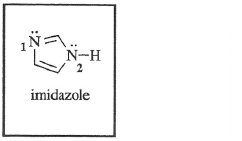# Problem: The imidazole ring system, which contains two nitrogen atoms, is found in the amino acid histidine. Which statement is true regarding the basicity of the two ring nitrogen atoms? A) Both are strongly basic since the ring is aromatic. B) The nitrogen atom labeled (1) is more basic than (2). C) The nitrogen atom labeled (2) is more basic than (1). D) Neither of the nitrogen atoms would be considered basic.

🤓 Based on our data, we think this question is relevant for Professor Paige's class at GMU.

###### Problem Details

The imidazole ring system, which contains two nitrogen atoms, is found in the amino acid histidine. Which statement is true regarding the basicity of the two ring nitrogen atoms?

A) Both are strongly basic since the ring is aromatic.

B) The nitrogen atom labeled (1) is more basic than (2).

C) The nitrogen atom labeled (2) is more basic than (1).

D) Neither of the nitrogen atoms would be considered basic.What scientific concept do you need to know in order to solve this problem?

Our tutors have indicated that to solve this problem you will need to apply the Basicity of Aromatic Heterocycles concept. You can view video lessons to learn Basicity of Aromatic Heterocycles. Or if you need more Basicity of Aromatic Heterocycles practice, you can also practice Basicity of Aromatic Heterocycles practice problems.

What is the difficulty of this problem?

Our tutors rated the difficulty ofThe imidazole ring system, which contains two nitrogen atoms...as medium difficulty.

How long does this problem take to solve?

Our expert Organic tutor, Jonathan took 2 minutes and 18 seconds to solve this problem. You can follow their steps in the video explanation above.

What professor is this problem relevant for?

Based on our data, we think this problem is relevant for Professor Paige's class at GMU.